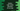# Python program to right rotate the elements of an array n number of times## Python program to right rotate the elements of an array n number of times:

In this post, we will learn how to right rotate or right shift an array for n number of times. Right rotation or right shift means moving all elements of an array towards right. The rightmost element moves to the start and other elements are moved to right.

### Right rotation or Right shift of array numbers:

Let’s take a look at the below image:

Here, we are rotating the array by 1 to the right. So, each elements are shifted by 1 to the right and the last element is moved to start.

Here, n is 1.

To rotate the array for any value of n, we can perform the above step for n number of times.

So,

• Loop through the array from right to left and shift all numbers by 1 position to its right.
• Move the last element to the start of the array.

### Python program:

Below is the complete python program:

``````def print_array(arr):
for i in range(0, len(arr)):
print(arr[i], end=' ')
print('\n')

given_arr = [1, 2, 3, 4, 5, 6, 7, 8, 9, 10]

print('Given Array :')
print_array(given_arr)

n = int(input('Enter the number of times for right rotation : '))

for i in range(0, n):
last_element = given_arr[-1]

for j in range(len(given_arr) - 1, -1, -1):
given_arr[j] = given_arr[j - 1]

given_arr = last_element

print_array(given_arr)``````

Here,

• print_array is used to print an array. This method takes an array and prints its value.
• We are taking the rotation number as input from the user and storing it in the variable n.
• The outer loop is used to make n number of rotation. On each iteration, it makes one rotation.
• The inner loop makes the rotation by 1. It moves all elements by one to the right and then the last element is placed at the first position. This way, for n iteration, it moves all elements by n.
• We are calling the print_array before and after the rotation. Since it changes the original array, we are printing the same array two times.

### Sample output:

If you run this program, it will print output as like below:

``````Given Array :
1 2 3 4 5 6 7 8 9 10

Enter the number of times for right rotation : 3
8 9 10 1 2 3 4 5 6 7

Given Array :
1 2 3 4 5 6 7 8 9 10

Enter the number of times for right rotation : 1
10 1 2 3 4 5 6 7 8 9 ``````